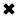# Electrical Resistance Explained#### The standard unit of resistance is the ohm

Electrical Resistance quantifies the opposition that a circuit imposes against the flow of electric current. You can compare resistance to the reciprocal of the diameter of a garden hose (where conductance compares to the actual diameter). For metal wire, this analogy works pretty well. Small-diameter wire has higher resistance than large-diameter wire made of the same metal.

The standard unit of resistance is the ohm, sometimes symbolized as an upper-case Greek letter omega (Q). You'll also hear about kilohms (symbolized k or kQ), where 1 k = 1000 ohms, or about megohms (symbolized M or MQ), where 1 M = 1,000,000 ohms = 1000 k. In this book, we'll never use the omega symbol. Instead, we'll always write out "ohm" or "ohms" in full.Table 2-1 shows the resistivity values for various common sizes of solid copper wire

Electric wire is sometimes rated for resistivity. The standard unit for this purpose is the ohm per foot (ohm/ft) or the ohm per meter (ohm/rn). You might also come across the unit ohm per kilometer (ohm/km). Table 2-1 shows the resistivity values for various common sizes of solid copper wire at room temperature, as a function of the wire size as defined by a scheme known as the American Wire Gauge (AWG).

When we place a potential difference of 1 V across a component whose resistance equals 1 ohm, assuming that the power supply can deliver an unlimited number of charge carriers, we get a current of 1A. If we double the resistance to 2 ohms, the current decreases to 0.5 A. If we cut the resistance by a factor of 5 to get only 0.2 ohms, the current increases by the same factor, from 1 A to 5 A. The current flow, for a constant voltage, varies in inverse proportion to the resistance. Figure 2-3 shows the current, through components of various resistances, given a constant potential difference of 1 V.Fig. 2-3 shows the current, through components of various resistances

Whenever an electric current flows through a component, a potential difference appears across that component. If the component has been deliberately manufactured to exhibit a certain resistance, we call it a resistor. Figure 2-4 illustrates this effect. In general, the potential difference arises in direct proportion to the current through the resistance. Engineers take advantage of this effect when they design electronic circuits.Fig. 2-4. Whenever current passes through a component having resistance, a voltage exists acrosss that component.

Electrical circuits always have some resistance. No such thing as a perfect conductor (an object with mathematically zero resistance) exists in the real world. When scientists cool certain metals down to temperatures near absolute zero, the substances lose practically all of their resistance, so that current can flow around and around for a long time. This phenomenon is called superconductivity. But nothing can ever become an absolutely perfect conductor. Such an object would in effect constitute a perpetual-motion machine.

Just as a perfectly resistance-free substance cannot exist in the real world, we'll never encounter an absolutely infinite resistance, either. Even dry air conducts electric current to some extent, although the effect is usually so small that scientists and engineers can ignore it. In some electronic applications, engineers select materials based on how "nearly infinite" their resistance appears; but when they say that, they exploit a figure of speech. They really mean to say that the resistance is so gigantic that we can consider it "infinite" for all practical purposes.

In electronics, the resistance of a component often varies, depending on the conditions under which that component operates. A transistor, for example, might have high resistance some of the time, and low resistance at other times. High/low resistance variations can take place thousands, millions or billions of times each second. In this way, oscillators, amplifiers, and digital devices function in radio receivers and transmitters, telephone networks, digital computers, and satellite links (to name just a few applications).

##### Basic Electricity Handbook, Vol. 1• FULLY ILLUSTRATED
• GREAT FOR SCHOOL PROJECTS
• GREAT PRICE: \$5.99
• ...

This 100+ page e-book is a great guide for those who have a basic interest in the field of electricity. This well-illustrated e-book, coupled with some basic knowledge of electricity, will give you a broad theoretical background in this fundamental subject.

CONTENTS
• FUNDAMENTALS OF ELECTRICITY
• HOW DOES ELECTRICITY WORK?
• HOW IS ELECTRICITY GENERATED?
• WHERE DOES ELECTRICITY COME FROM?
• BASIC HOME WIRING
• ELECTRICITY FOR STUDENTS
• ENERGY SAVING TIPS

##### TRAINING EF COURSES

Content Community Connection
Top